If you’re looking for a vertex distance conversion charts, you’ve found the motherload. Here you will find vertex distance conversion charts for single powers (i.e Sphere power only), as well as for astigmatism (Sphere + Cylinder combinations).

## Sphere Power Only

Lets start your typical Sphere Power Only Vertex Distance Conversion Chart.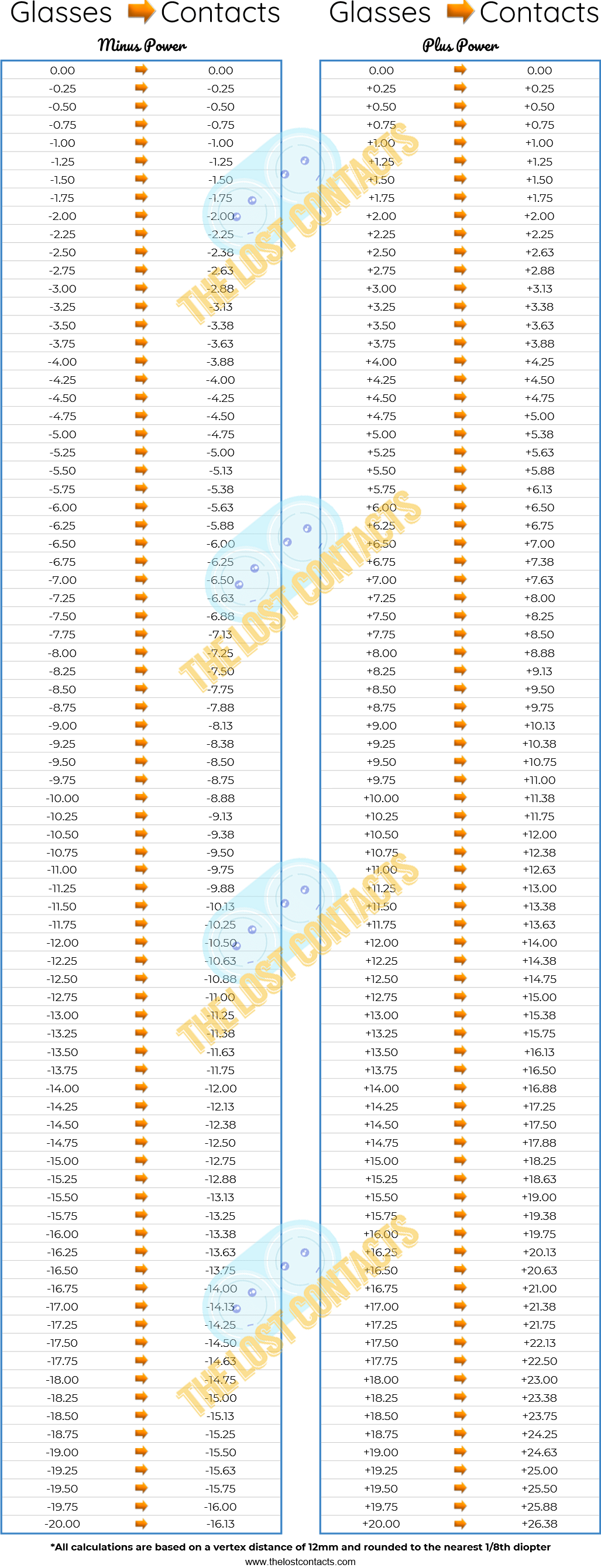If you are looking you convert a combination of Sphere and Cylinder powers, you will need to reference the following Vertex Distance Conversion Chart for Astigmatism.

## Astigmatism Charts For Minus Sphere Powers

This series of charts will cover all Sphere and Cylinder combinations ranging from Sphere Power 0 to -10.75 and Cylinder Power 0 to -4.00.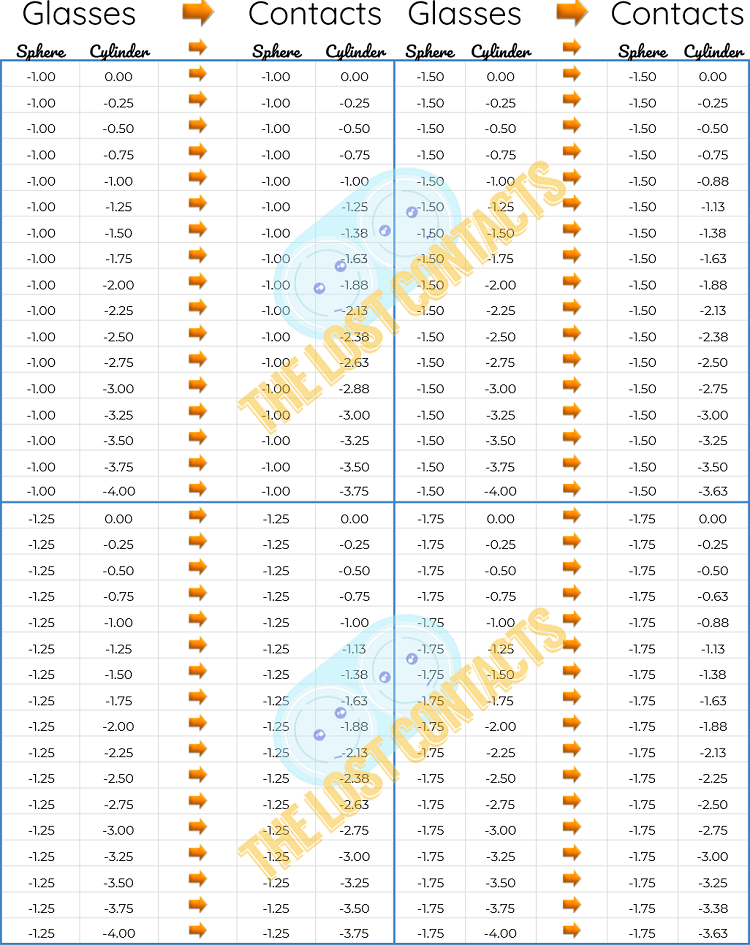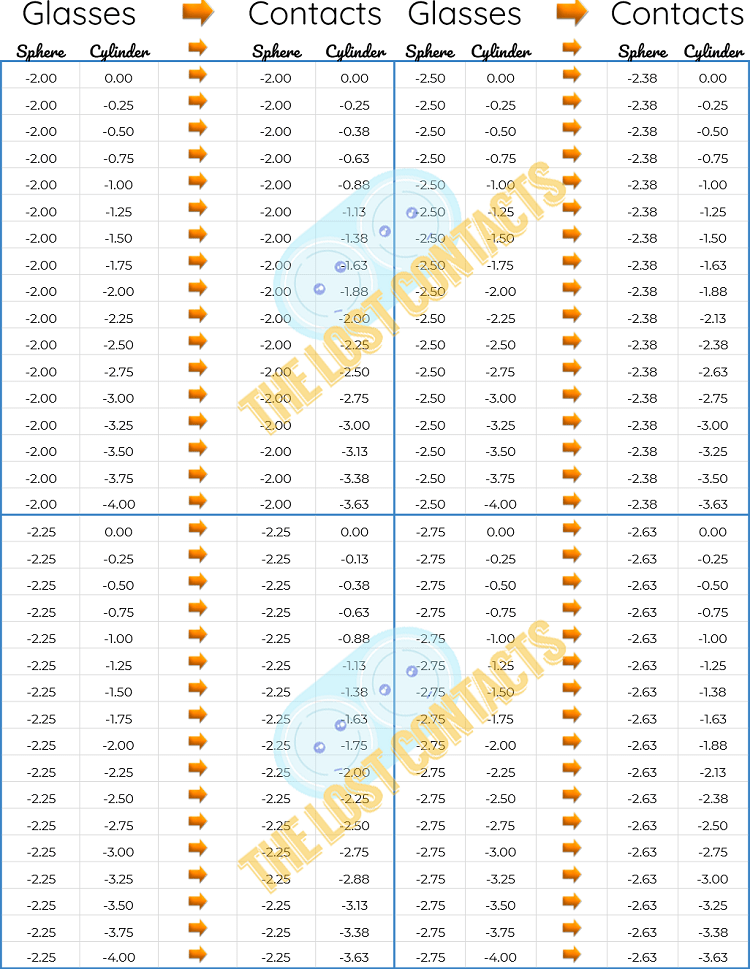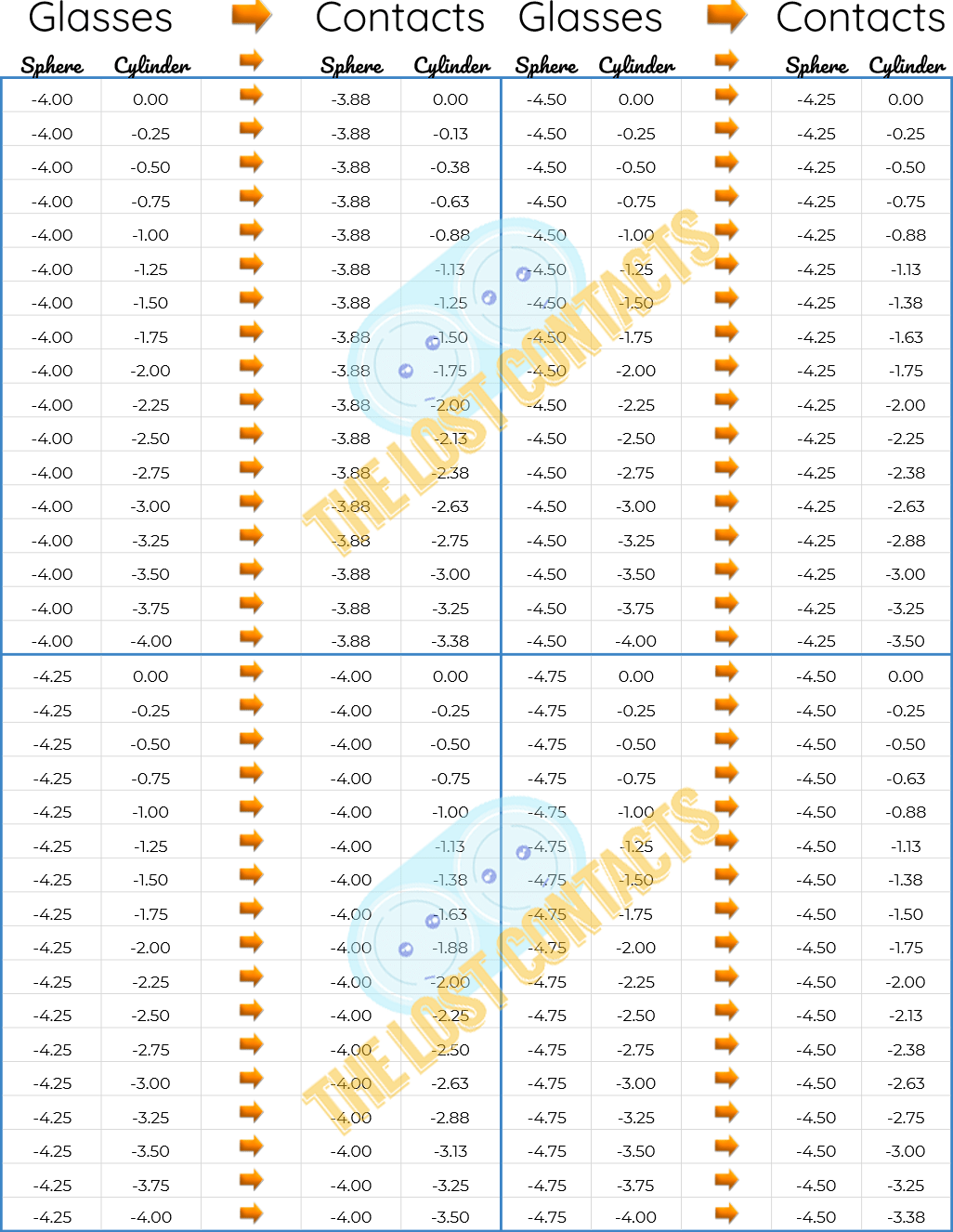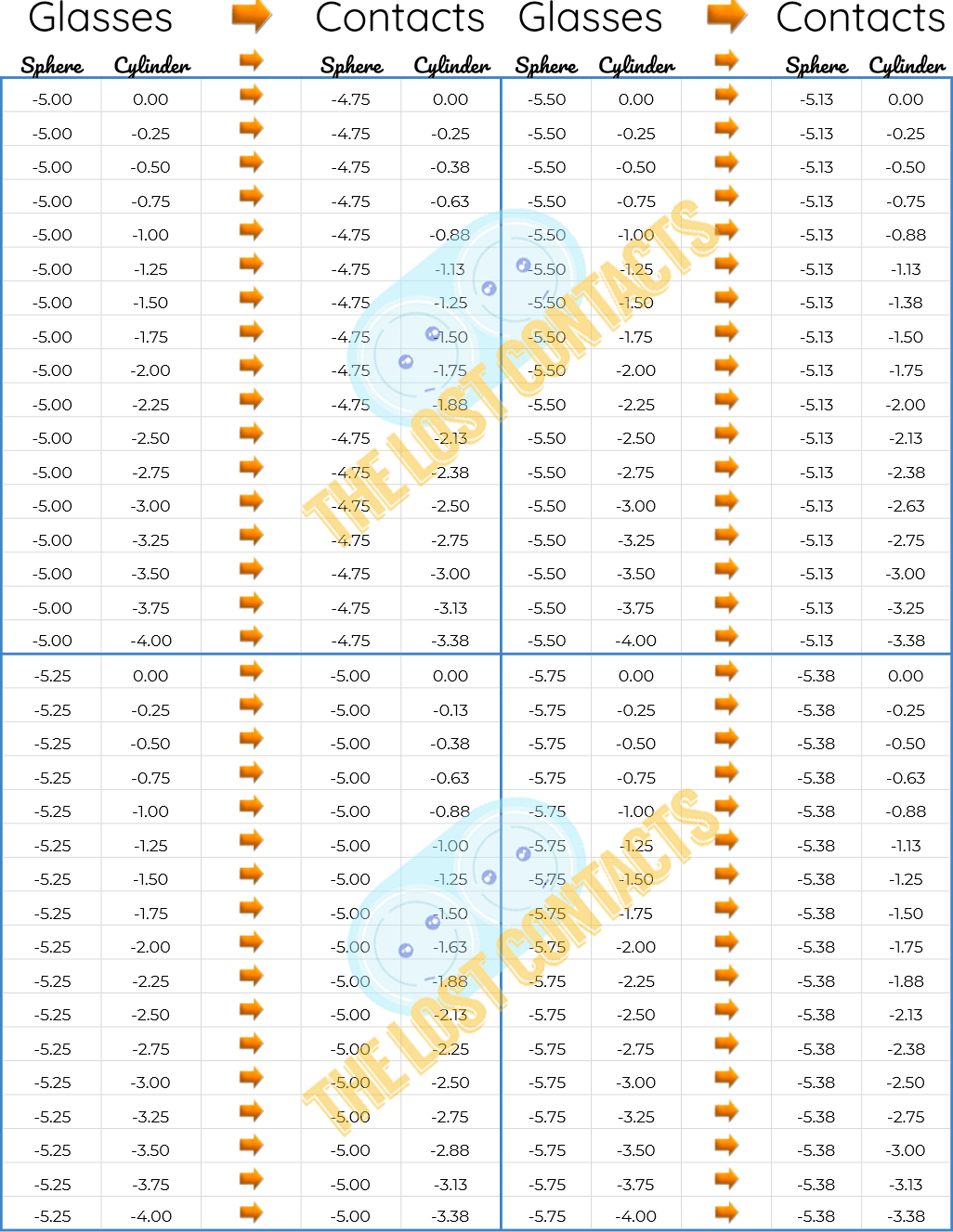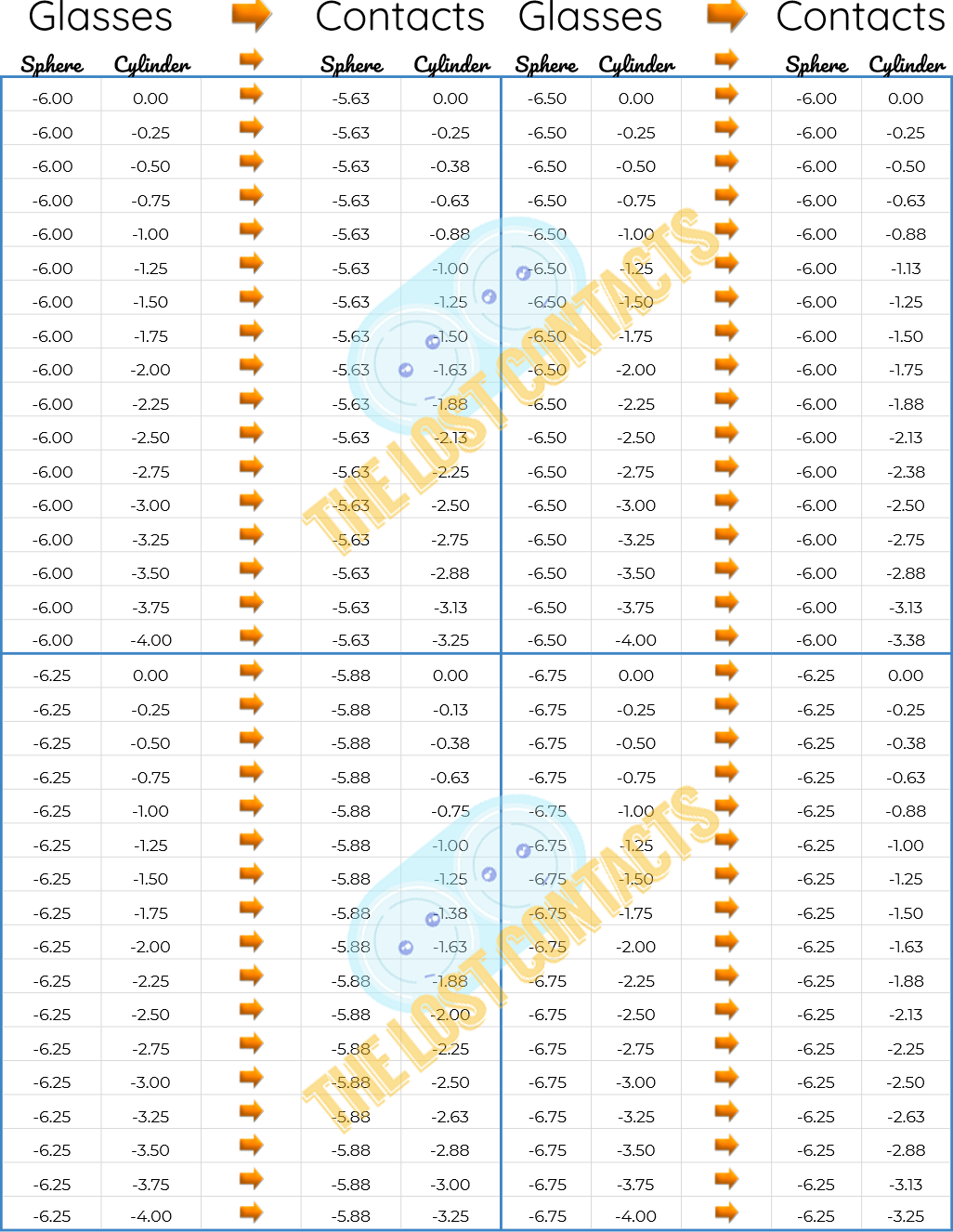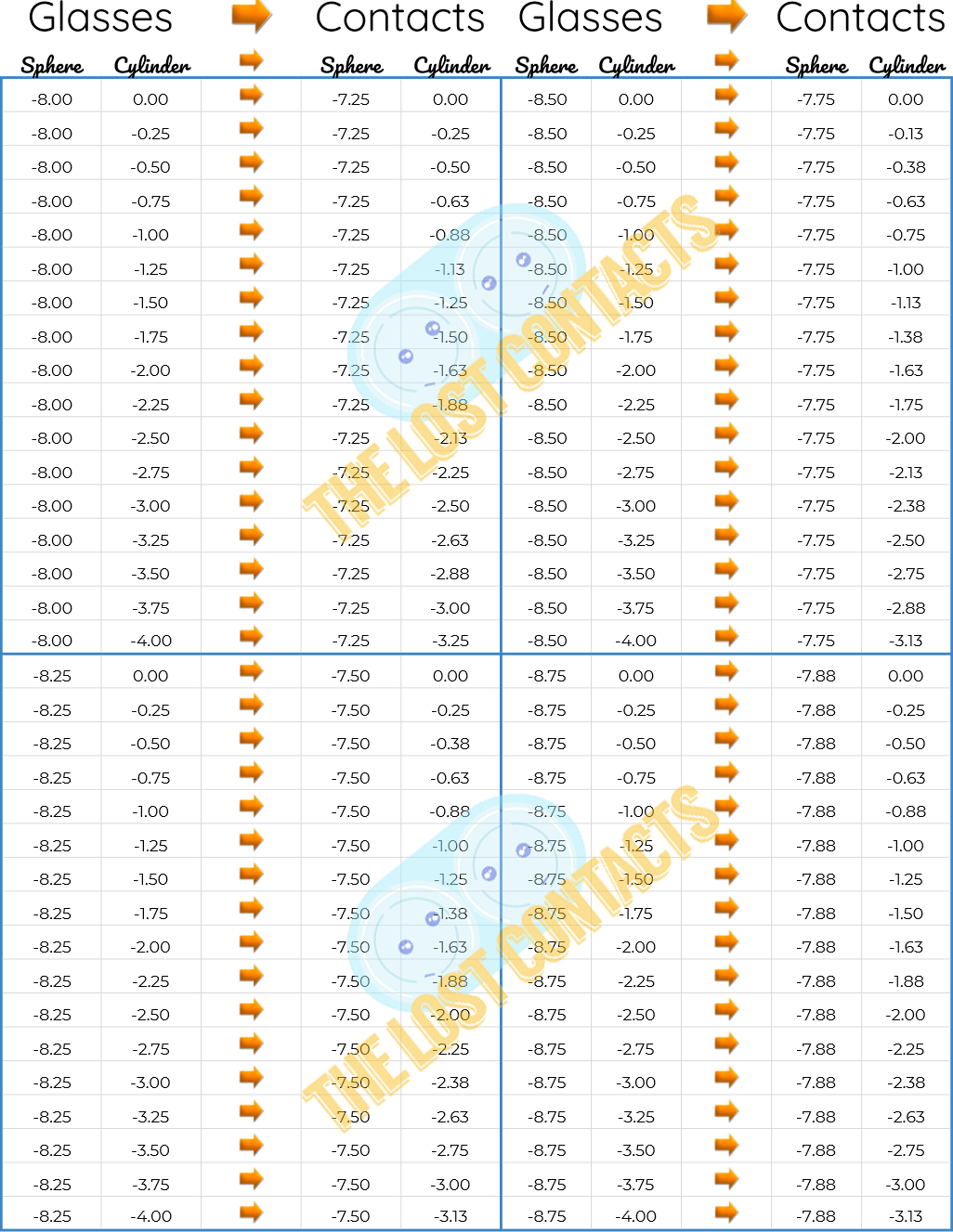## Astigmatism Charts For Plus Sphere Powers

The next series of charts will cover all Sphere and Cylinder combinations ranging from Sphere Power 0 to +10.75 and Cylinder Power 0 to -4.00.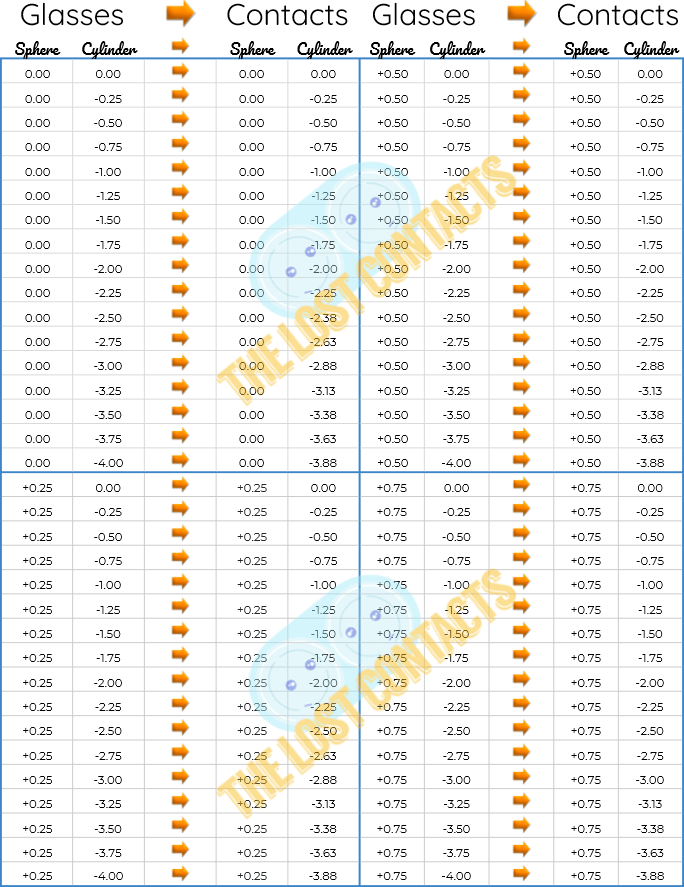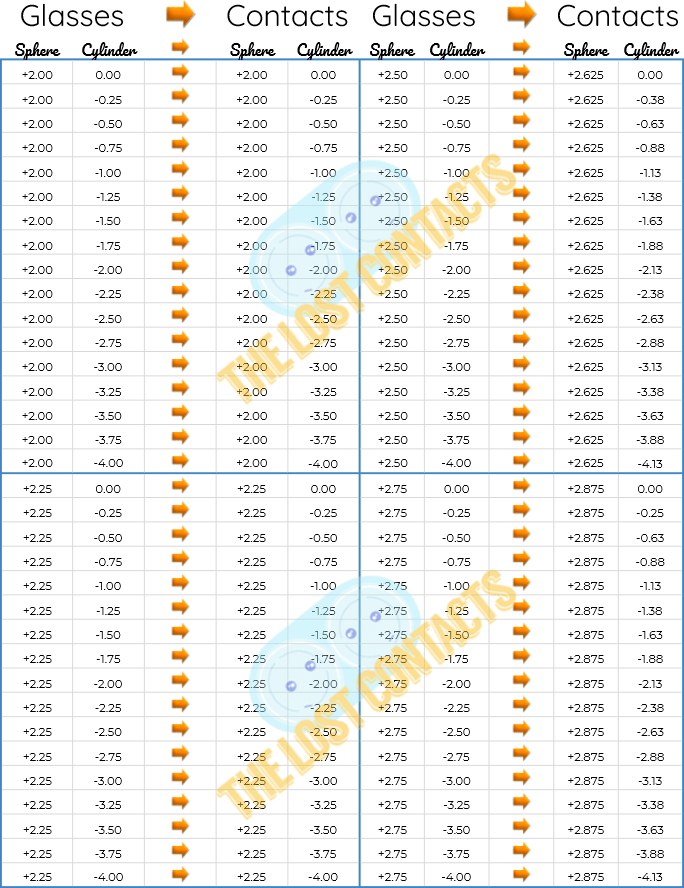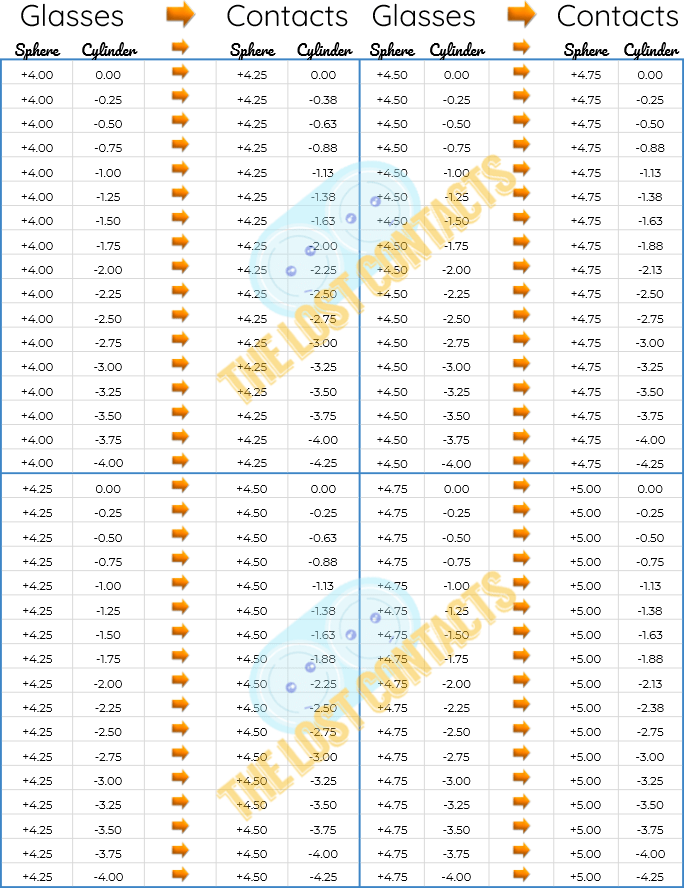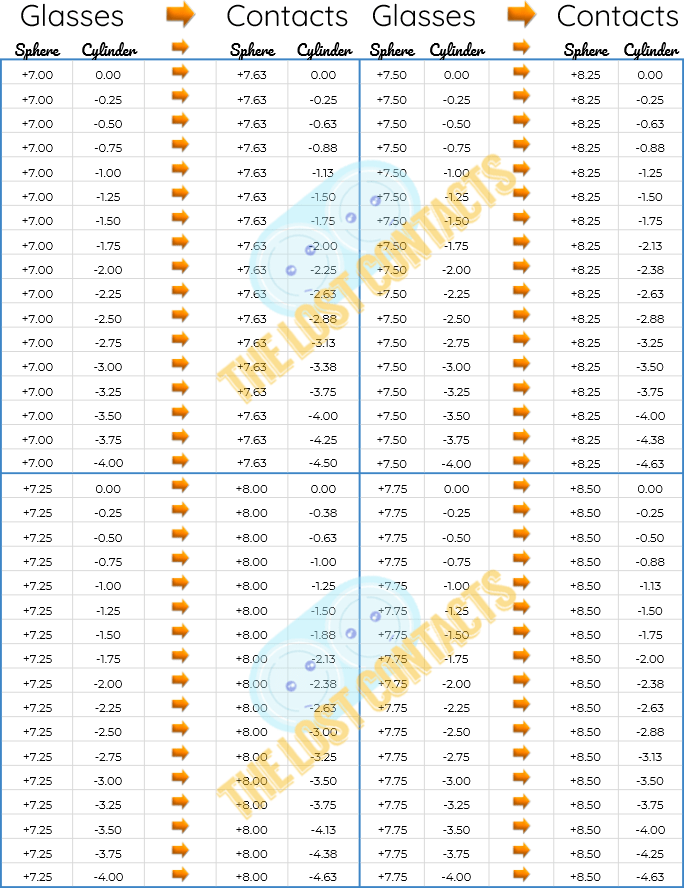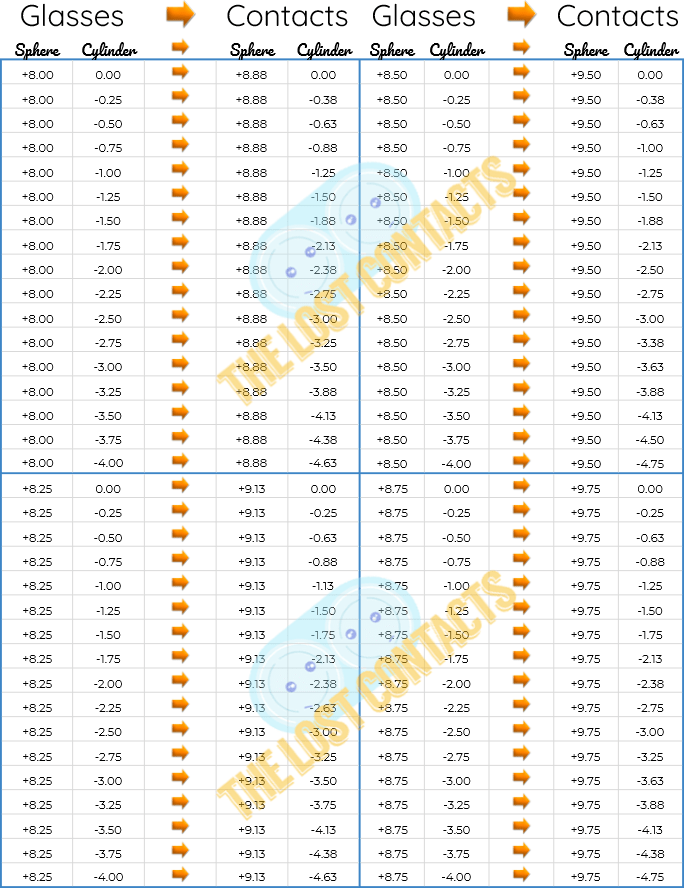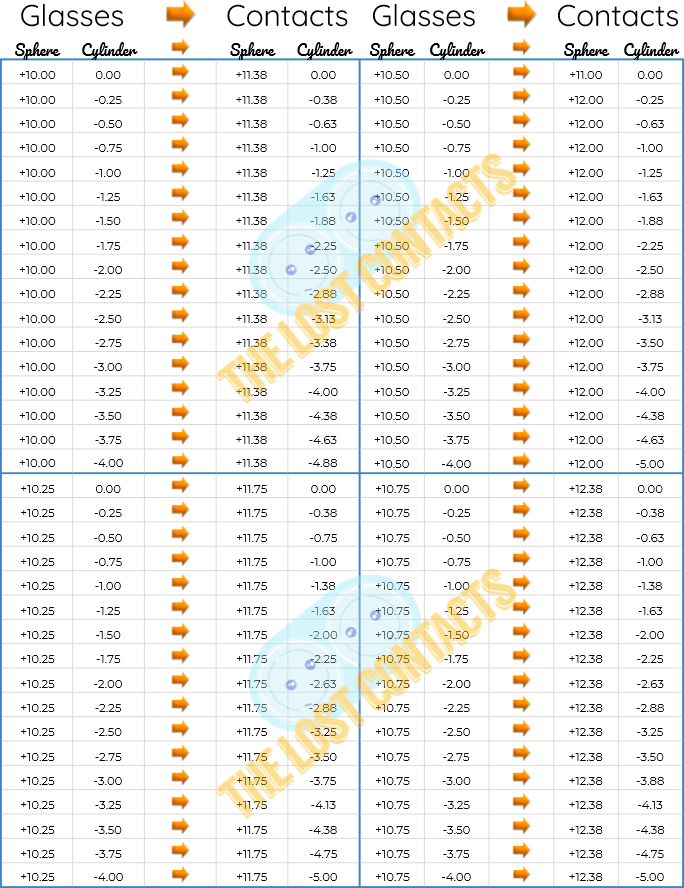## How Were These Charts Calculated?

All the charts were populated using the following equation: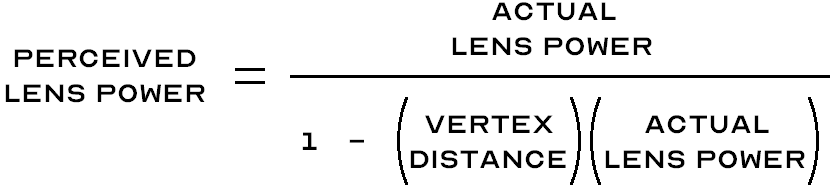The vertex distance used in every calculation was 12mm.

## Summary

I hope these charts provide value for you and save you time in your clinic. If you would like to download and print these charts, you can get the pdfs in The Lost Contacts downloads section.